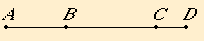# Proposition 79

To an apotome only one rational straight line can be annexed which is commensurable with the whole in square only.
X.73

Let AB be an apotome, and BC an annex to it. Then AC and CB are rational straight lines commensurable in square only.

I say that no other rational straight line can be annexed to AB which is commensurable with the whole in square only.X.73

If possible, let BD be so annexed. Then AD and DB are also rational straight lines commensurable in square only.

II.7

Now, since the excess of the sum of the squares on AD and DB over twice the rectangle AD by DB is also the excess of the sum of the squares on AC and CB over twice the rectangle AC by CB, for both exceed by the same, the square on AB, therefore, alternately, the excess of the sum of the squares on AD and DB over the sum of the squares on AC and CB is the excess of twice the rectangle AD by DB over twice the rectangle AC by CB.

But the sum of the squares on AD and DB exceeds the sum of the squares on AC and CB by a rational area, for both are rational, therefore twice the rectangle AD by DB also exceeds twice the rectangle AC by CB by a rational area, which is impossible, for both are medial, and a medial area does not exceeded a medial by a rational area.

Therefore no other rational straight line can be annexed to AB which is commensurable with the whole in square only.

Therefore only one rational straight line can be annexed to an apotome which is commensurable with the whole in square only.

Therefore, to an apotome only one rational straight line can be annexed which is commensurable with the whole in square only.

Q.E.D.

## Guide

This proposition is used in X.81 and X.84.